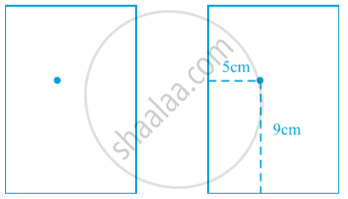Share

# Introduction of Coordinate Geometry

#### notes

The definition of coordinate geometry is the study of algebraic equations on graphs. A coordinate geometry is a branch of geometry where the position of the points on the plane is defined with the help of an ordered pair of numbers also known as coordinates.
For example :
Suppose you put a dot on a sheet of paper in following first fig.The position of the dot on the paper, how will you do this? The dot is in the upper half of the paper”, or “It is near the left edge of the paper”, or “It is very near the left hand upper corner of the sheet”.  The dot is nearly 5 cm away from the left edge of the paper”, it helps to give some idea but still does not fix the position of the dot. . A little thought might enable you to say that the dot is also at a distance of 9 cm above the bottom line.
For this purpose, we fixed the position of the dot by specifying its distances from two fixed lines, the left edge of the paper and the bottom line of the paper in above second Fig. In other words, we need two independent informations for finding the position of the dot.

### Shaalaa.com

Coordinate Geometry [00:16:47]
S
0%

S